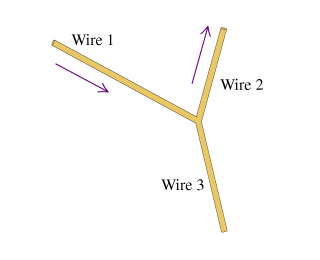# Problem: Consider the juncion of three wires as shown in the diagram. (Figure 1)The magnitudes of the current density and the diameters for wires 1 and 2 are given in the table. The current directions are indicated by the arrows.WireCurrent density(A/mm2)Diameter(mm)13.11.624.92.8Find the current I3 in wire 3.Express your answer in amperes to two significant figures. Call current out of the junction positive and current into the junction negative.

###### FREE Expert Solution

Kirchhoff's junction rule:

$\overline{){\mathbf{\Sigma }}{{\mathbf{I}}}_{\mathbf{i}\mathbf{n}}{\mathbf{=}}{\mathbf{\Sigma }}{{\mathbf{I}}}_{\mathbf{o}\mathbf{u}\mathbf{t}\mathbf{}}}$

I1 + I3 = I2

Current density, J = I/A

I = JA

86% (190 ratings)###### Problem Details

Consider the juncion of three wires as shown in the diagram. (Figure 1)The magnitudes of the current density and the diameters for wires 1 and 2 are given in the table. The current directions are indicated by the arrows.

 Wire Current density(A/mm2) Diameter(mm) 1 3.1 1.6 2 4.9 2.8

Find the current I3 in wire 3.

Express your answer in amperes to two significant figures. Call current out of the junction positive and current into the junction negative.